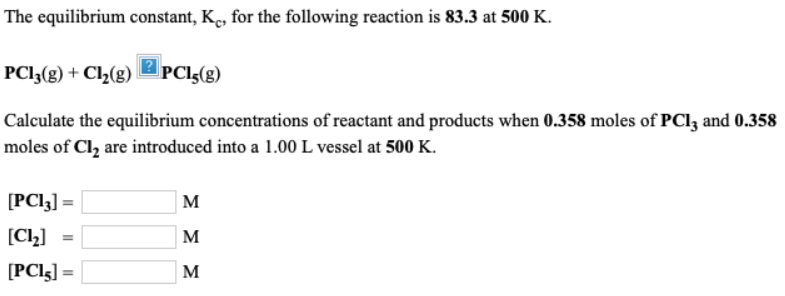# The equilibrium constant, Kc, for the following reaction is 83.3 at 500 K. PCl3(g) + Cl2(g) -> PCl5(g) Calculate the equilibrium concentrations of reactant and products when 0.358 moles of PCl3 and 0.358 moles of Cl2 are introduced into a 1.00 L vessel at 500 K. [PCl3] = ___ M [Cl2] = ___ M [PCl5] = ___ M